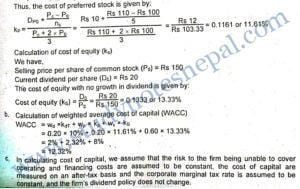###### 1. What is the significance of marginal cost of capital in decision making? Explain.

Marginal cost of capital is the cost of raising an additional rupee of capital. In other words, it is the cost incurred for raising incremental funds. Thus, the weighted average cost of incremental capital is known as the marginal cost of capital. The concept of marginal cost of capital is significant in all financial decisions. They are described as below:

• Use in investment decision: In evaluating the profitability of long-term investment alternatives, the financial manager uses the marginal cost of capital as the standard. If the investment alternative cannot generate returns at least equal to marginal cost of capital, it is not profitable.
• Use in financing decision: The marginal cost of capital concept also plays an important role in designing the optimal capital structure of a firm. When a firm is going to raise additional capital, should it use debt or equity or mix of these sources? This decision is undertaken considering the impact of financing decision on marginal cost of capital.
• Use in dividend decision: The concept marginal cost of capital is also useful in dividend policy decision. Dividend distribution affects the amount of internal financing available for reinvestment to the firm. Whether a firm should retain the earnings for reinvestment or should rely on external funds is decided considering their impact on marginal cost of capital.
###### 2. What are the factors affecting cost of capital? Explain.

Various factors affect a firm’s cost of capital. They are discussed as follows:

• General economic conditions: General economic conditions affect the demand and supply of capital in the economy. If demand for capital increases, without corresponding increase in supply, the cost of capital increases, and vice versa.
• Marketability: Marketability of the securities also affects the cost of capital. For example, investors require a higher rate of return from investment in less marketable securities which pushes up the cost of capital.
• Financing need: Higher the amount of financing needed of a firm higher will be the I weighted average cost of capital. For example, a higher amount of financing leads to higher flotation costs, under pricing of the security, discount and so on, thus increasing the cost of capital.
• Operating and financial risk: Operating risk is the variability in returns on assets, which is affected by the company’s investment decisions. Financial risk is the increased variability in returns to common stockholders resulted from using fixed charge bearing sources of financing. As these risks increase, the cost of capital also increases.
• Other factors: Besides above, other factors such as tax rate, capital structure and dividend policy also affect the cost of capital. For example, higher tax rate reduces the cost of debt, which ultimately reduces the cost of capital; use of more debt decreases WACC for a given level of cost of equity: higher dividend payout ratio increases the amount of external equity financing and ultimately increases the WACC.
###### 3. What are the various ways the cost of retained earnings can be estimated?

The cost of retained earnings is the minimum required rate of return that a firm must earn on its internal equity. Retained earnings are created by forgoing dividend payment to stockholders. There is no direct or explicit cost associated with the use of retained earnings. The cost of retained earnings is implicit. It is the opportunity cost of dividend income foregone by stockholders. The cost of retained earnings can be estimated in the following ways:

(a) Discounted cash flow method: According to this method, the cost of retained earnings is the sum of expected dividend yield and growth rate. But if growth rate is not constant, the trial-and-error approach is used to compute the cost of retained. For a constant growth stock, cost of retained earnings is given by:

kr=D₁ /Po + g

(b) Capital Asset Pricing Model (CAPM): The CAPM model describes the relationship between the non-diversifiable risk of the firm and cost of common stock. According to this model, the cost of internal equity is equal to risk-free rate plus appropriate risk premium for the level of risk involved. It is calculated using following equation:

kr = RF + [E(RM) – RF]

(c) Bond-yield-plus-risk-premium method: According to this method, the cost of internal equity can be determined by simply adding a risk premium of three to five percentage points to the cost of the firm’s own long-term debt. This is stated as follows:

Kr = Bond yield + Risk premium

###### 4. Marginal cost of capital.

Marginal cost of capital is the cost of raising an additional rupee of capital. In other words, it is the weighted average cost of incremental capital. So long as a firm maintains the same capital structure, its cost of raising additional debt stays at the same level, and it satisfies all new equity requirements with retained earnings, the marginal cost of capital remains equal to the weighted average cost of the capital employed by the firm. However, the marginal cost of capital does not remain constant at all times. As the volume of new financing increases, the costs of the various sources of financing also increase. For example, if a firm raises new debt, the suppliers of debt capital may require higher returns in the form of increased interest rate to compensate for the additional financial risk brought about by the use of additional debt. Similarly, after a certain limit of new financing, the retained earnings may not be sufficient to satisfy the equity requirement of new financing. In this case, the firm has to approach external equity by issuing new shares. While selling new shares to raise additional equity, the firm may have to under-price the issues and incur flotation costs, which increase the cost of equity. These factors combined together push up the marginal cost of capital.

The rupee amount of total new financing beyond which the marginal cost of capital increases is called break point. In other words, the break point is the rupee volume of new capital that can be raised before an increase in the firm’s weighted average marginal cost of capital. The increase in cost of any source of new financing causes a break in marginal cost of capital. In other words, the break in marginal cost of capital appears due to increased cost of new equity or increased cost of new debt or both.

## Numerical Problems

The Morang Soap Company’s next expected dividend is Rs.3.18; its growth rate is 6 percent; and the stock now sells for Rs.36. New stock can be sold to net the firm Rs.32.40 per share.

a. What is the company’s percentage flotation cost?

b. What is the company’s cost of new common stock?

SOLUTION

We have,

Expected dividend per share (D₁) = Rs 3.18

Growth rate (g) = 6%

Market price of stock per share (Po) = Rs 36

Net proceed per share from new stock (NP) = Rs 32.40

a. Percentage floatation cost is given by:

Percentage flotation cost (f) = Po-NP / Po

=Rs 36 -32.40 / Rs36

= 0.10 or 10

b. Cost of new common stock is given by:

Kn = D₁ / NP + g

=Rs 3.18 / Rs 32.40  +0.06

= 0.1581 or 15.81%

Cost of new common stock is the cost of raising equity by selling additional shares of common stock externally. Selling of new common stock involves flotation costs, therefore the cost of new equity is always higher than the cost of internal equity.

The Jhapa Rice Mill’s cost of equity is 16 percent. Its before-tax cost of debt is 13 percent, and its average tax rate is 40 percent. The stock sells at book value. Using the following balance sheet, calculate company’s after-tax weighted average cost of capital: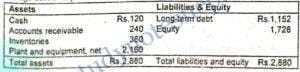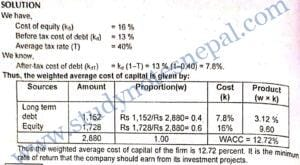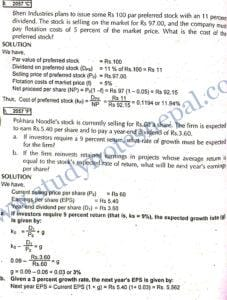On January 1, 20X1, International Copy Machines (ICOM), one of the favorites of the stock market, was priced at Rs 300 per share. This price was based on an expected dividend at the end of the year of Rs.3 per share and an expected annual growth rate in dividends of 20 percent into the future. By January 20X2, economic indicators have turned down, and investors have revised their estimate for future dividend growth of ICOM downward to 15 percent. What should be the price of the firm’s common stock in January 20X2? Assume the following:

(a) A constant dividend growth valuation model is a reasonable representation of the way the market values ICOM.

(b) The firm does not change the risk complexion of its assets nor its financial leverage. (c) The expected dividend at the end of 20X2 is Rs 3.45 pers share.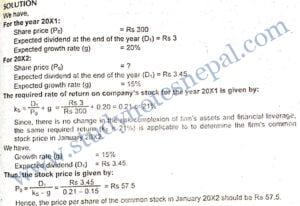PP Corporation has a target capital structure of 40 percent debt and 60 percent common equity. The company’s before tax cost of debt is 12 percent and its marginal tax rate is 40 percent. The current stock price is Po = Rs. 22.50; the last dividend was Do Rs. 2.00; and the dividend is expected to grow at a constant rate of 7 percent. What will be the firm’s cost of common equity and its WACC? What will happen to WACC if PP Corporation needs to spend flotation cost on the issue of common stock? Explain.

SOLUTION

We have,

Proportion of debt (wd) = 40%

Proportion of equity (ws) = 60%

Before tax cost of debt (kd) = 12%

Marginal tax rate (T) = 40%

Current stock price (Po) = Rs 22.50

The last dividend (Do) = Rs 2

Growth rate (g) = 7%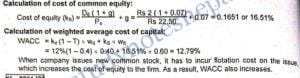Pashupati Electric Company (PEC) uses only debt and equity. It can borrow unlimited amounts at an interest rate of 10 percent as long as it finances at its target capital structure, which calls for 45 percent debt and 55 percent common equity. Its last dividend was Rs. 2, its expected constant growth rate is 4 percent, and its stock sells at a price of Rs. 20. PEC’s tax rate is 40 percent. Two projects are available: Project A has a rate of return of 13 percent, while Project B has a rate of return of 10 percent. All of the company’s potential projects are equally risky and as risky as the firm’s other assets.

a. What is PEC’s cost of common equity?

b. What is PEC’s weighted average cost of capital?

c. Which projects should PEC select?

SOLUTION

We have,

Before tax cost of debt (kd) = 10%

Proportion of debt (wd) = 45%

Proportion of equity (ws) = 55%

Last dividend per share (Do) = Rs 2

Growth rate (g) = 4%

Current selling price per share (Po) = Rs 20

Tax rate (T) = 40%

IRR of Project A = 13%

IRR of Project B= 10%.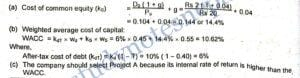The following tabulation gives earnings per share figures for the K and K Company during the preceding 5 years. The firm’s common stock, 6 million shares outstanding, is now (1/1/2004) selling for Rs. 70 per share, and the expected dividend at the end of the current year 2004 is 60 percent of the 2003 EPS. Because investors expect the past trend to continue, g may be based on the earnings growth rate.

Calculate:

a. Company’s after tax cost of new debt and of common equity, assuming that new equity comes only from retained earnings.

b. Company’s weighted average cost of capital, again assuming that present capital structure is maintained.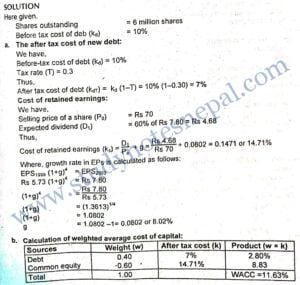A firm has planned to raise the required fund from the following sources:

(i) Selling bond at par, with 10 percent coupon rate.

(ii) Selling preferred stock at par value of Rs 100 with a 10 % coupon and a call price of Rs.110, if the firm plans to call the issue in 5 years.

(iii) Selling Rs 100 par value common stock at Rs.150 and paying a Rs.20 dividend, which is expected to be continued indefinitely.

Required:

(a) Compute the component cost of each source of financing.

(b) Compute the weighted average cost of capital of the firm if the firm has 20 % debt and 20% preference stock, and the balance common stock.

(c) What are the assumptions of cost of capital?

SOLUTION

1. Calculation of after tax cost of bond (kdT) Since the corporate marginal tax rate is not given we assume the tax rate is zero percent. In the absence of taxes, if bonds sell at par and there is no flotation cost associated with bond issue, the cost of debt is equal to its coupon rate. Thus, After-tax cost of debt (kdT) = Before tax cost (1-T) = 10% (1 – 0) = 10%.

Calculation of cost of preferred stock (kp) We have,

Selling price per share of preferred stock (Po) = Rs 100 Preferred stock dividend per share (Dps) = Par value x Dividend rate = Rs 100 × 0.10 = Rs 10

Call price (Pc) = Rs 110

Call period (nc) = 5 years